1. Home
2. â€º
3. Blog
4. â€º

# Biopharmaceutical calculations - AUC and clearance

by egpat       08-06-2017

Biopharmaceutical calculations are very important in order to determine the amount of drug reaches to systemic circulation and to fix the dose and dosing intervals.

When drugs are given orally they should undergo absorption into the systemic circulation. Drugs cann't absorb completely due to various factors like physicochemical properties of the drugs, physiological factors and even first pass metabolism.

The total amount of the drug that reaches into the systemic circulation is called as bioavailable dose.

Calculation of fraction of bioavailability

Working example 1: F From dose

400 mg of a drug is reached in to the systemic circulation when given at dose of 500 mg. Calculate the fraction of  drug reached in to the systemic circulation.

Solution:

Now let’s see the solution for the above example.

Data given: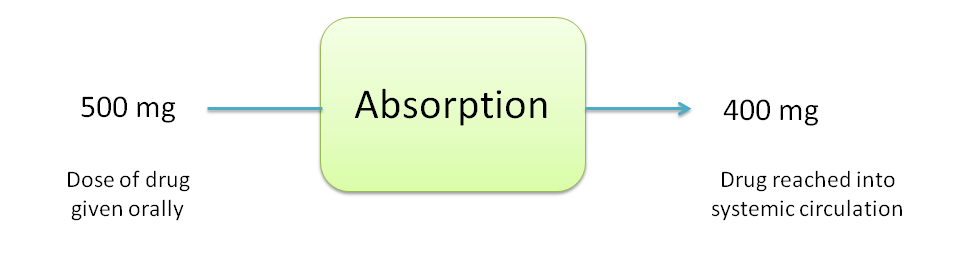Oral dose=500 mg

Bioavailble dose=400 mg

Fraction of drug absorbed=?

The fraction of the dose absorbed can be calculated as follows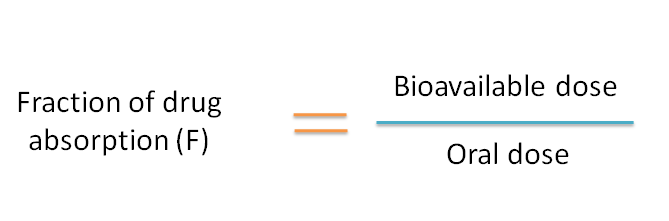By using above equation,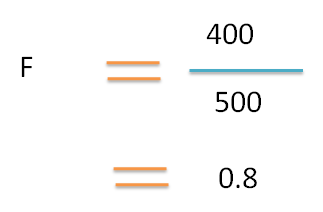Working example 2: F From AUC

The fraction of the drug reaching in to the systemic circulation for a 500 mg of drug with clearance 20 L/hr is given produced AUC 10 mgL-1 hr

Solution:

First of let's define AUC and clearance

AUC

AUC is the Area Under Curve which indicates the rate and extent of systemic absorption of the drug. The total amount the drug absorbed into the systemic circulation is directly proportional to AUC.

Clearance

We have to use the equation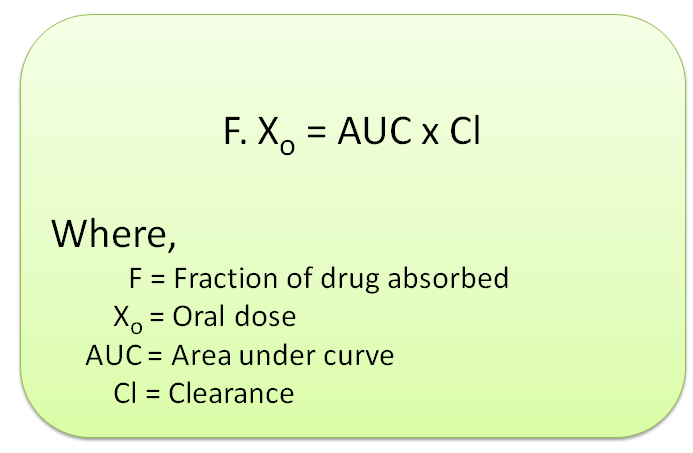Clearance is related with volume of distribution as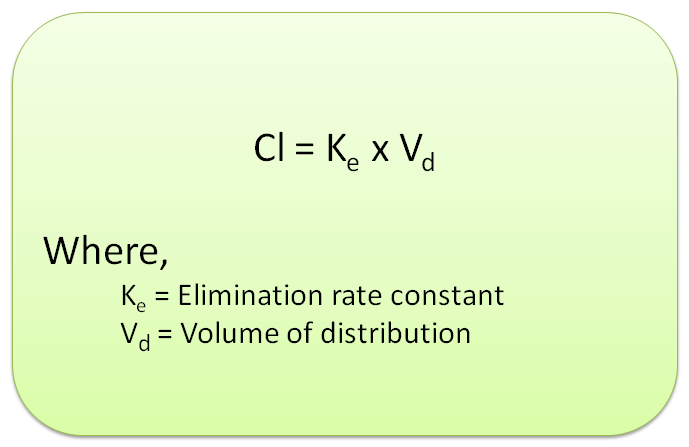Hence combining above two equation, we can bring a final equation.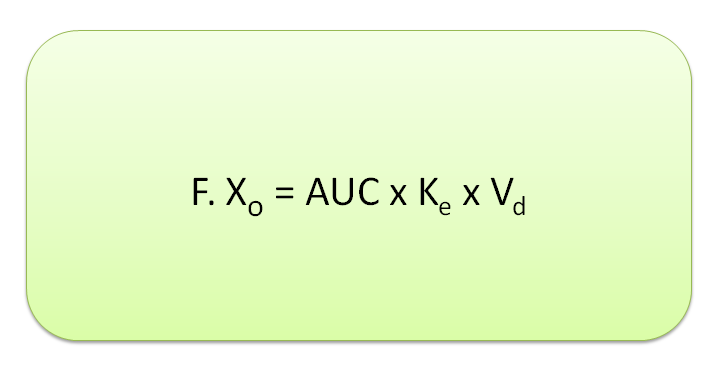Data given:

Dose, Xo=500mg

Clearance, Cl=20 L/hr

AUC=10 mgL-1 hr

What is required

Fraction of dose absorbed=?

By using above equation,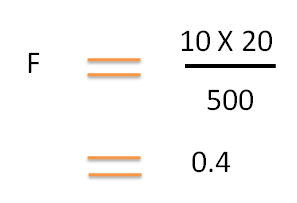Working Example 3:  AUC from Clearance

The AUC of a 400 mg of drug given by oral route having 10 L/hr clearance, if 90% absorbed is

Data given :

Dose=400 mg

Clearance=10 L/hr

Fraction of dose absorbed=90%=0.9

AUC=?

Again using the above equation,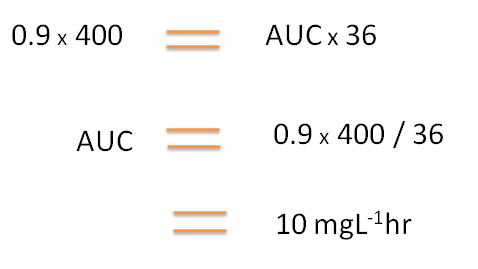Working Example 4:  Dose for given AUC

How much dose of a drug is required to achieve AUC . The clearance of the drug is and drug is 80% absorbed.

Data given :

Clearance=10 L/hr

Fraction of dose absorbed=80%=0.8

AUC=40 mgL-1 hr

What is required

Dose=?

Again using the above equation,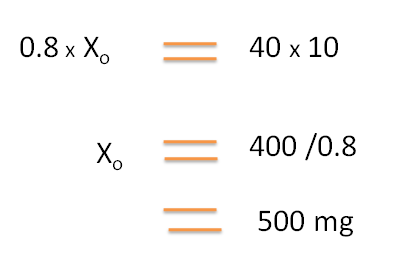Working Example 5: Dose for given clearance

Calculate dose of a drug with elimination rate constant 0.8 hr-1 that is required to administer to attain AUC of  25 mgL-1 hr . The volume of distribution of the drug is 20L.

Data given :

Elimination rate constant, Ke=0.8 hr-1

AUC=25 mgL-1 hr

Volume of distribution, Vd=20 L

Fraction of dose absorbed=1

What is required

Dose=?